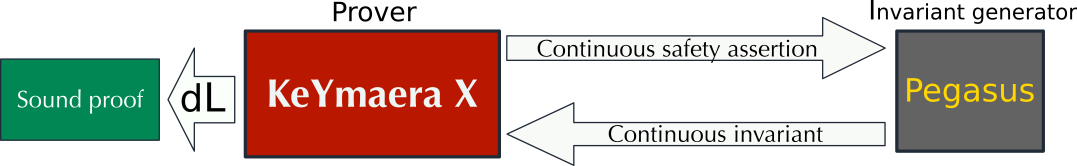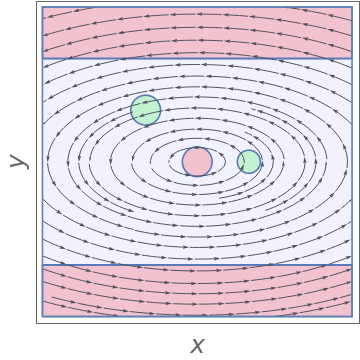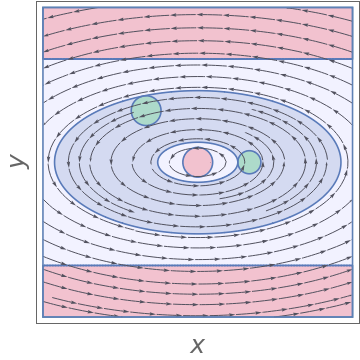# A tool for generating inductive invariants for continuous systems

Pegasus is a tool for generating continuous invariants for systems described by polynomial ordinary differential equations. It is integrated with the KeYmaera X theorem prover for hybrid systems and implements a number of methods for invariant generation. Pegasus provides a framework for combining techniques for invariant generation into more powerful strategies. Its ultimate purpose is to improve the level of proof automation in KeYmaera X and other deductive verification frameworks for hybrid systems.# System requirements and installation guidelines

In order to use Pegasus, one needs to have access to Mathematica and MATLAB, as well as a working installation of KeYmaera X.

### Installation instructions

Pegasus is implemented in the Wolfram Language and runs inside Mathematica; it is (optionally) able to make use of MATLAB for additional computations. The simplest way to get started is:

• Ensure Mathematica version 10+ is installed on your system.
• Download and follow the installation instructions for KeYmaera X. Pegasus works out of the box with KeYmaera X once Mathematica is configured properly.
• Ensure MATLAB version 2016+ is installed on your system.
• Run the following in Mathematica:

SystemOpen@FileNameJoin[{\$UserBaseDirectory, "Applications"}]

and extract the contents of the downloaded MATLink into the folder opened by the above command (on Unix-like systems this folder is usually /home/.Mathematica/Applications, but the value of \$UserBaseDirectory varies on different systems; see documentation).

• Run the following in Mathematica:

OpenMATLAB[]

• Go to http://matlink.org/troubleshooting/ for troubleshooting if the above commands return errors.

• Download SeDuMi and SOSTOOLS (on MacOS use tbxmanager to install SeDuMi; otherwise there is a missing dependency to some native .dylib),
• Unzip and add all subfolders to the MATLAB path by navigating to the MATLAB window menu and selecting Home -> Set Path. Use "Add with Subfolders" and select the folders containing the unzipped SeDuMi and SOSTOOLS files, e.g.

Make sure to save your changes in order to add these folders to the path permanently.

# Running with KeYmaera X

Pegasus ships with KeYmaera X and is used automatically in some parts of the proof automation. In order to discover the right proof and controllers you can also use Tools→Find ODE Invariant Candidates. Keep in mind that the version of Pegasus that ships with KeYmaera X gets better when installing the additional software such as MATLab etc.

# Running Pegasus separately

Using Pegasus as a standalone tool can be achieved by navigating to keymaerax-core/src/main/resources/Pegasus inside the folder containing the KeYmaera X source directory. The file Pegasus.m serves as the main package from which invariant generation strategies may be called. Various examples of invariant generation using this file are contained in the Tests/ sub-folder. Keep in mind that by running Pegasus separately using Mathematica one only obtains invariant candidates, whereas KeYmaera X additionally formally proves the invariance property of these candidates.

In Mathematica, an input problem for Pegasus takes the form of a continuous Hoare triple:

{precondition, {vector_field, state_variables, evolution_constraint}, postcondition}

where precondition, postcondition and evolution_constraint are first-order formulas of real arithmetic, state_variables is a finite list of the system's state variables (e.g. {x,y,z}), and vector_field is a list of equal size containing polynomials in those variables (corresponding to the right-hand side of the system of ODEs). For instance, when calling from a notebook file in the Test/ sub-folder in the Pegasus main directory:

 In = SetDirectory[ParentDirectory[NotebookDirectory[]]] In = Needs["Pegasus`", FileNameJoin[{Directory[], "Pegasus.m"}]] In = InvGen[{(-2 + x)^2 + y^2 <= 1/5 || (2 + x)^2 + (-2 + y)^2 <= 1/3, {{-4 y, x}, {x, y}, True}, y <= 4 && y >= -4 && x^2 + y^2 > 1/3}]

In the illustration of this verification problem below, the unsafe sets are shown in light red and the initial states in light green:The output is a nested list, whose first formal entry is the invariant candidate (other entries in the list serve to facilitate proof construction within KeYmaera X and may be ignored when using Pegasus separately).

Out = {{106345581/44105831 < x^2+4 y^2 < 784012261/25449817,{{x^2+4 y^2 < 784012261/25449817,kyx`ProofHint==kyx`FirstIntegral},{x^2+4 y^2 > 106345581/44105831,kyx`ProofHint==kyx`FirstIntegral}}},True}

When drawn, the invariant looks as follows (shown in light blue):